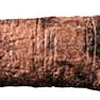#### You may also like### Where Can We Visit?

Charlie and Abi put a counter on 42. They wondered if they could visit all the other numbers on their 1-100 board, moving the counter using just these two operations: x2 and -5. What do you think?### Babylon Numbers

Can you make a hypothesis to explain these ancient numbers?### Ishango Bone

Can you decode the mysterious markings on this ancient bone tool?

# Arithmagons

### Why do this problem?

This problem offers students the opportunity to explore number patterns with or without the use of symbols, and offers several routes to generality.

Relating to this month's theme, think of the process of putting numbers in the vertices and then calculating the edge numbers as an action. Is it possible to undo that action uniquely, that is, to 'solve' the arithmagon?

### Possible approach

Suggest that the students watch you in silence and then describe what you have done.
Draw the skeleton of an arithmagon, fill in numbers at the vertices and then the numbers on the edges.
Do this again, completing the numbers at the vertices and waiting to be prompted by the class before completing the numbers on the edges. You could introduce fractions, decimals and/or negative numbers.

Once you are convinced that they know what's happening, draw another skeleton and fill in the numbers on the edges. Can they find the numbers at the vertices?

"Do you think you will be able to complete any arithmagon if I give you the numbers on the edges?"

"Try it out yourselves - draw a skeleton and choose some numbers to go on the edges. Can you now work out the numbers that must go at the vertices?"

If a computer room is available, students could work on the problem using the interactivity.

Any arithmagon they can't complete could be displayed for whole class consideration.

After some time draw the class together and share findings. The following questions could be used as a focus for discussion:

• Are there any arithmagons that you haven't been able to complete?
• When do you need negative numbers?
• When do you need fractions or decimals?
• Does anyone have a strategy for completing the arithmagons?
• Can algebra help us?
Further questions worth considering can be found at the end of the problem.

It might be interesting to ask students to try Sums of Pairs a few weeks later and see if they notice that it is essentially the same problem.

### Key questions

Is it always possible to find numbers to go at the vertices given any three numbers on the edges?

What is the relationship between the total of the edge numbers and the total of the vertex numbers?

### Possible support

To help students get started, use the interactivity on Level 1 to generate arithmagons which can be solved using only positive whole numbers.

### Possible extension

Investigate the properties of the quadrilateral arithmagons generated by putting two arithmagons side by side overlapping along one edge.

Investigate square arithmagons.
Can all square arithmagons be solved? Are the solutions unique?

Students might also like to explore the related concept of magic graphs .

Here are some NRICH magic graph challenges:

Magic W
Olympic Magic